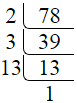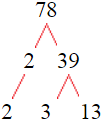# Factors of 78The factors of 78 are 1, 2, 3, 6, 13, 26, 39, and 78 i.e. F78 = {1, 2, 3, 6, 13, 26, 39, 78}. The factors of 78 are all the numbers that can divide 78 without leaving a remainder.

We can check if these numbers are factors of 78 by dividing 78 by each of them. If the result is a whole number, then the number is a factor of 78. Let's do this for each of the numbers listed above:

·        1 is a factor of 78 because 78 divided by 1 is 78.

·        2 is a factor of 78 because 78 divided by 2 is 39.

·        3 is a factor of 78 because 78 divided by 3 is 26.

·        6 is a factor of 78 because 78 divided by 6 is 13.

·        13 is a factor of 78 because 78 divided by 13 is 6.

·        26 is a factor of 78 because 78 divided by 26 is 3.

·        39 is a factor of 78 because 78 divided by 39 is 2.

·        78 is a factor of 78 because 78 divided by 78 is 1.

## How to Find Factors of 78?

1 and the number itself are the factors of every number. So, 1 and 78 are two factors of 78. To find the other factors of 78, we can start by dividing 78 by the numbers between 1 and 78. If we divide 78 by 2, we get a remainder of 0. Therefore, 2 is a factor of 78. If we divide 78 by 3, we get a remainder of 0. Therefore, 3 is also a factor of 78.

Next, we can check if 4 is a factor of 78. If we divide 78 by 4, we get a remainder of 2. Therefore, 4 is not a factor of 78. We can continue this process for all the possible factors of 78.

Through this process, we can find that the factors of 78 are 1, 2, 3, 6, 13, 26, 39, and 78. These are the only numbers that can divide 78 without leaving a remainder.

********************

********************

## Properties of the Factors of 78

The factors of 78 have some interesting properties. One of the properties is that the sum of the factors of 78 is equal to 168. We can see this by adding all the factors of 78 together:

1 + 2 + 3 + 6 + 13 + 26 + 39 + 78 = 168

Another property of the factors of 78 is that the prime factors of 78 are 2, 3, and 13 only.

## Applications of the Factors of 78

The factors of 78 have several applications in mathematics. One of the applications is in finding the highest common factor (HCF) of two or more numbers. The HCF is the largest factor that two or more numbers have in common. For example, to find the HCF of 78 and 65, we need to find the factors of both numbers and identify the largest factor they have in common. The factors of 78 are 1, 2, 3, 6, 13, 26, 39, and 78. The factors of 65 are 1, 5, 13, and 65. The largest factor that they have in common is 13. Therefore, the HCF of 78 and 65 is 13.

Another application of the factors of 78 is in prime factorization. Prime factorization is the process of expressing a number as the product of its prime factors. The prime factors of 78 are 2, 3, and 13 since these are the only prime numbers that can divide 78 without leaving a remainder. Therefore, we can express 78 as:

78 = 2 × 3 × 13

We can do prime factorization by division and factor tree method also. Here is the prime factorization of 78 by division method,78 = 2 × 3 × 13

Here is the prime factorization of 78 by the factor tree method,78 = 2 × 3 × 13

## Conclusion

The factors of 78 are the numbers that can divide 78 without leaving a remainder. The factors of 78 are 1, 2, 3, 6, 13, 26, 39, and 78. The factors of 78 have some interesting properties, such as having a sum of 168. The factors of 78 have several applications in mathematics, such as finding the highest common factor and prime factorization.